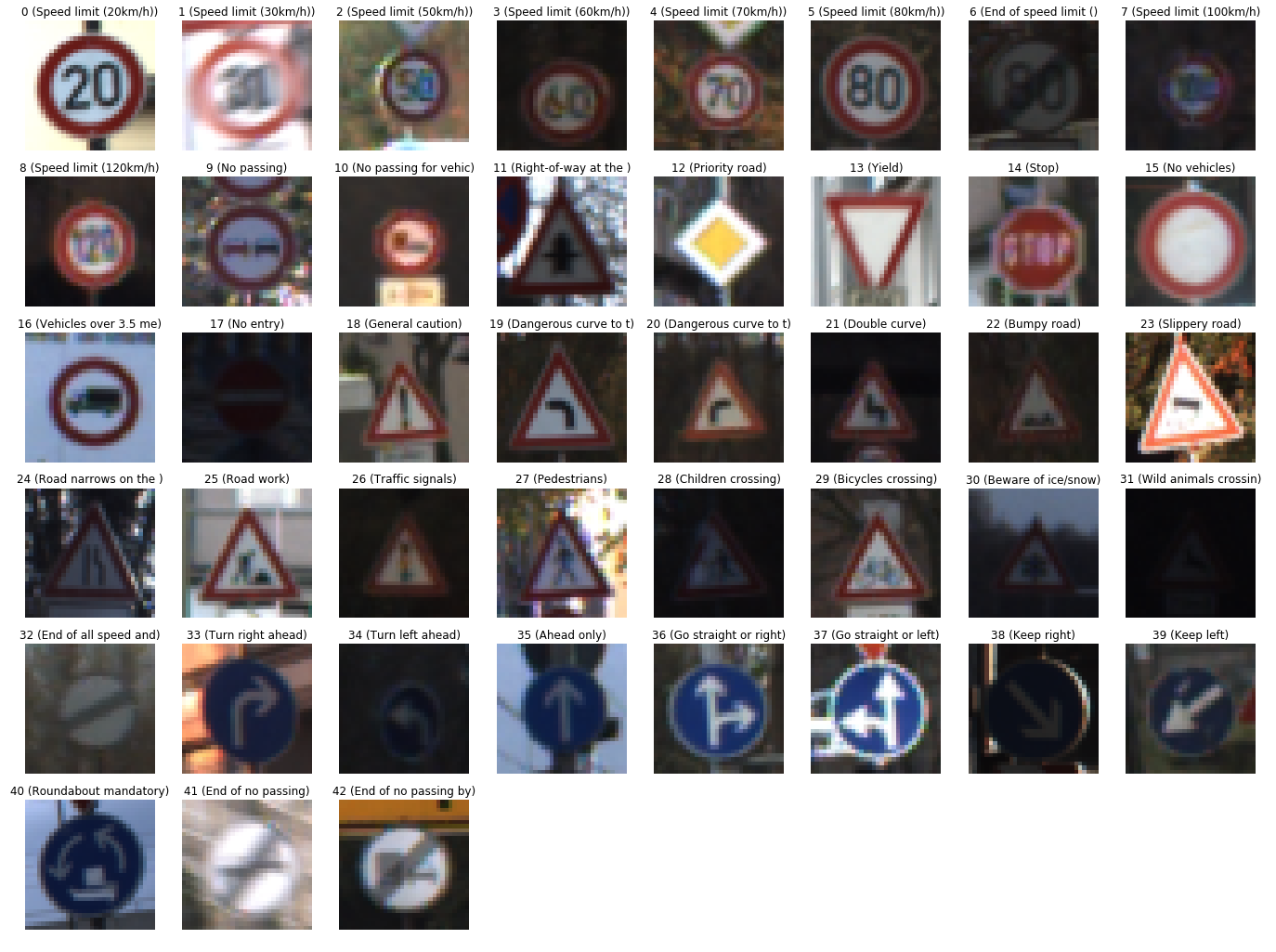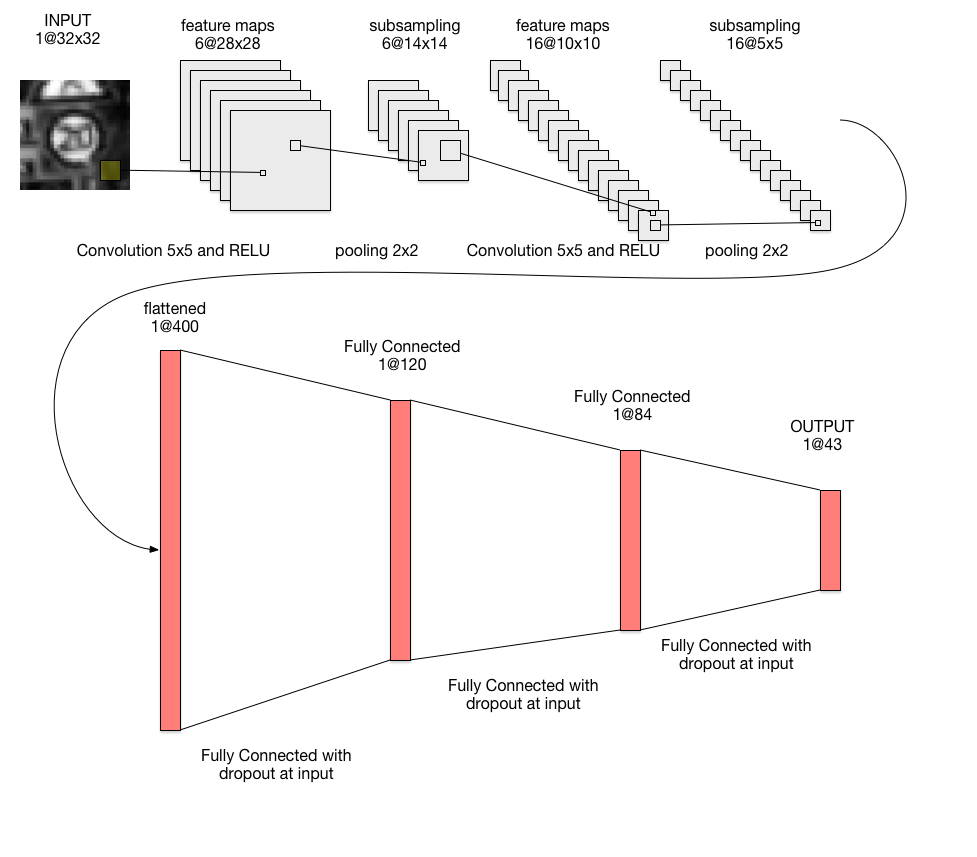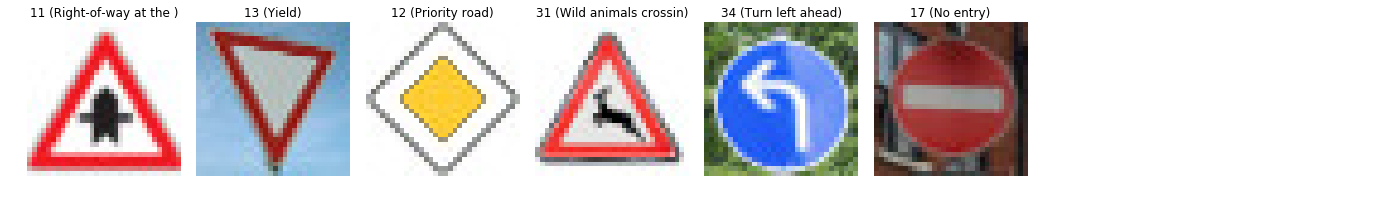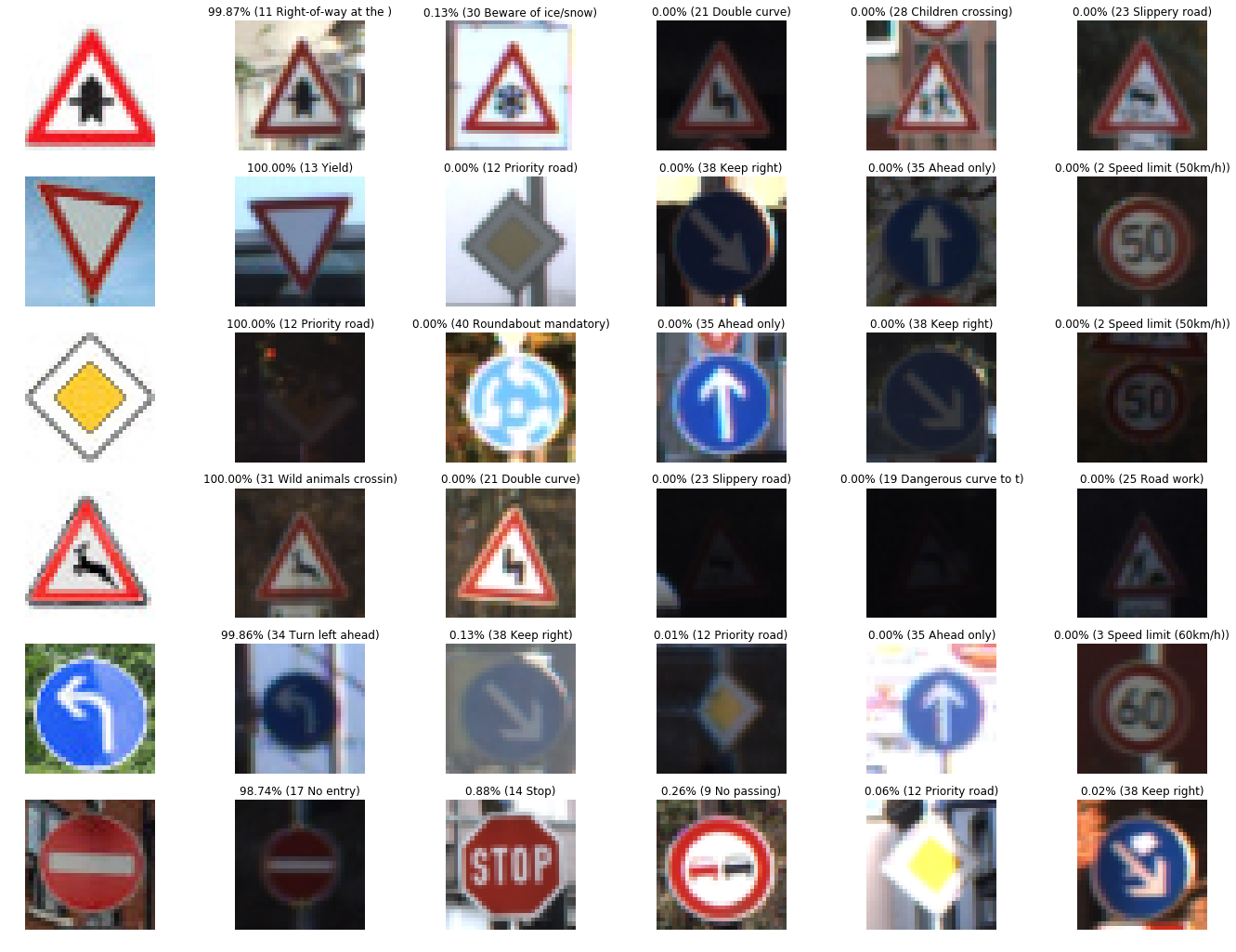# Traffic Sign Classifier

Udacity Self-Driving Car Nanodegree

View the Project on GitHub MarkBroerkens/CarND-Traffic-Sign-Classifier-Project

Abstract

This project shows how to classify german traffic signs using a modified LeNet neuronal network. (See e.g. Yann LeCu - Gradiant-Based Learning Applied to Document Recognition)

The steps of this project are the following:

• Load the data set (see below for links to the project data set)
• Explore, summarize and visualize the data set
• Design, train and test a model architecture
• Use the model to make predictions on new images
• Analyze the softmax probabilities of the new images
• Summarize the results with a written report

## Rubric Points

### Submitted Files

1. README.md that includes all the rubric points and how I addressed each one. You’re reading it!
2. The jupyter notebook
3. HTML output of the code

### Data Set Summary & Exploration

#### 1. Dataset Summary

I used the numpy library to calculate summary statistics of the traffic signs data set:

• The size of training set is 34799
• The size of the validation set is 4410
• The size of test set is 12630
• The shape of a traffic sign image is (32, 32, 3)
• The number of unique classes/labels in the data set is 43

#### 2. Exploratory Visualization

The following figure shows one example image for each label in the training set.Here is an exploratory visualization of the data set. It is a bar chart showing how many samples are contained in the training set per label.### Design and Test a Model Architecture

#### 1. Preprocessing

As a first step, I decided to convert the images to grayscale because several images in the training were pretty dark and contained only little color und the grayscaling reduces the amount of features and thus reduces execution time. Additionally, several research papers have shown good results with grayscaling of the images. Yann LeCun - Traffic Sign Recognition with Multi-Scale Convolutional Networks

Here is an example of a traffic sign image before and after grayscaling.Then, I normalized the image using the formular `(pixel - 128)/ 128` which converts the int values of each pixel [0,255] to float values with range [-1,1]

#### 2. Model Architecture

The model architecture is based on the LeNet model architecture. I added dropout layers before each fully connected layer in order to prevent overfitting. My final model consisted of the following layers:

Layer Description
Input 32x32x1 gray scale image
Convolution 5x5 1x1 stride, valid padding, outputs 28x28x6
RELU
Max pooling 2x2 stride, outputs 14x14x6
Convolution 5x5 1x1 stride, valid padding, outputs 10x10x16
RELU
Max pooling 2x2 stride, outputs 5x5x16
Flatten outputs 400
Dropout
Fully connected outputs 120
RELU
Dropout
Fully connected outputs 84
RELU
Dropout
Fully connected outputs 43
Softmax#### 3. Model Training

To train the model, I used an Adam optimizer and the following hyperparameters:

• batch size: 128
• number of epochs: 150
• learning rate: 0.0006
• Variables were initialized using the truncated normal distribution with mu = 0.0 and sigma = 0.1
• keep probalbility of the dropout layer: 0.5

My final model results were:

• training set accuracy of 99.6%
• validation set accuracy of 97.5%
• test set accuracy of 95.1%

#### 4. Solution Approach

I used an iterative approach for the optimization of validation accuracy:

1. As an initial model architecture the original LeNet model from the course was chosen. In order to tailor the architecture for the traffic sign classifier usecase I adapted the input so that it accepts the colow images from the training set with shape (32,32,3) and I modified the number of outputs so that it fits to the 43 unique labels in the training set. The training accuracy was 83.5% and my test traffic sign “pedestrians” was not correctly classified. (used hyper parameters: EPOCHS=10, BATCH_SIZE=128, learning_rate = 0,001, mu = 0, sigma = 0.1)

2. After adding the grayscaling preprocessing the validation accuracy increased to 91% (hyperparameter unmodified)

3. The additional normalization of the training and validation data resulted in a minor increase of validation accuracy: 91.8% (hyperparameter unmodified)

4. reduced learning rate and increased number of epochs. validation accuracy = 94% (EPOCHS = 30, BATCH_SIZE = 128, rate = 0,0007, mu = 0, sigma = 0.1)

5. overfitting. added dropout layer after relu of final fully connected layer: validation accuracy = 94,7% (EPOCHS = 30, BATCH_SIZE = 128, rate = 0,0007, mu = 0, sigma = 0.1)

6. still overfitting. added dropout after relu of first fully connected layer. Overfitting reduced but still not good

7. added dropout before validation accuracy = 0.953 validation accuracy = 95,3% (EPOCHS = 50, BATCH_SIZE = 128, rate = 0,0007, mu = 0, sigma = 0.1)

8. further reduction of learning rate and increase of epochs. validation accuracy = 97,5% (EPOCHS = 150, BATCH_SIZE = 128, rate = 0,0006, mu = 0, sigma = 0.1)### Test a Model on New Images

#### 1. Acquiring New Images

Here are some German traffic signs that I found on the web:The “right-of-way at the next intersection” sign might be difficult to classify because the triangular shape is similiar to several other signs in the training set (e.g. “Child crossing” or “Slippery Road”). Additionally, the “Stop” sign might be confused with the “No entry” sign because both signs have more ore less round shape and a pretty big red area.

#### 2. Performance on New Images

Here are the results of the prediction:The model was able to correctly guess 5 of the 5 traffic signs, which gives an accuracy of 100%. This compares favorably to the accuracy on the test set of 95.1%

The code for making predictions on my final model is located in the 21th cell of the jupyter notebook.

#### 3. Model Certainty - Softmax Probabilities

In the following images the top five softmax probabilities of the predictions on the captured images are outputted. As shown in the bar chart the softmax predictions for the correct top 1 prediction is bigger than 98%.The detailed probabilities and examples of the top five softmax predictions are given in the next image.### Possible Future Work

#### 1. Augmentation of Training Data

Augmenting the training set might help improve model performance. Common data augmentation techniques include rotation, translation, zoom, flips, inserting jitter, and/or color perturbation. I would use OpenCV for most of the image processing activities.

#### 2. Analyze the New Image Performance in more detail

All traffic sign images that I used for testing the predictions worked very well. It would be interesting how the model performs in case there are traffic sign that are less similiar to the traffic signs in the training set. Examples could be traffic signs drawn manually or traffic signs with a label that was not defined in the training set.

#### 3. Visualization of Layers in the Neural Network

In Step 4 of the jupyter notebook some further guidance on how the layers of the neural network can be visualized is provided. It would be great to see what the network sees. Additionally it would be interesting to visualize the learning using TensorBoard

#### 4. Further Experiments with TensorFlow

I would like to investigate how alternative model architectures such as Inception, VGG, AlexNet, ResNet perfom on the given training set. There is a tutorial for the TensorFlow Slim library which could be a good start.